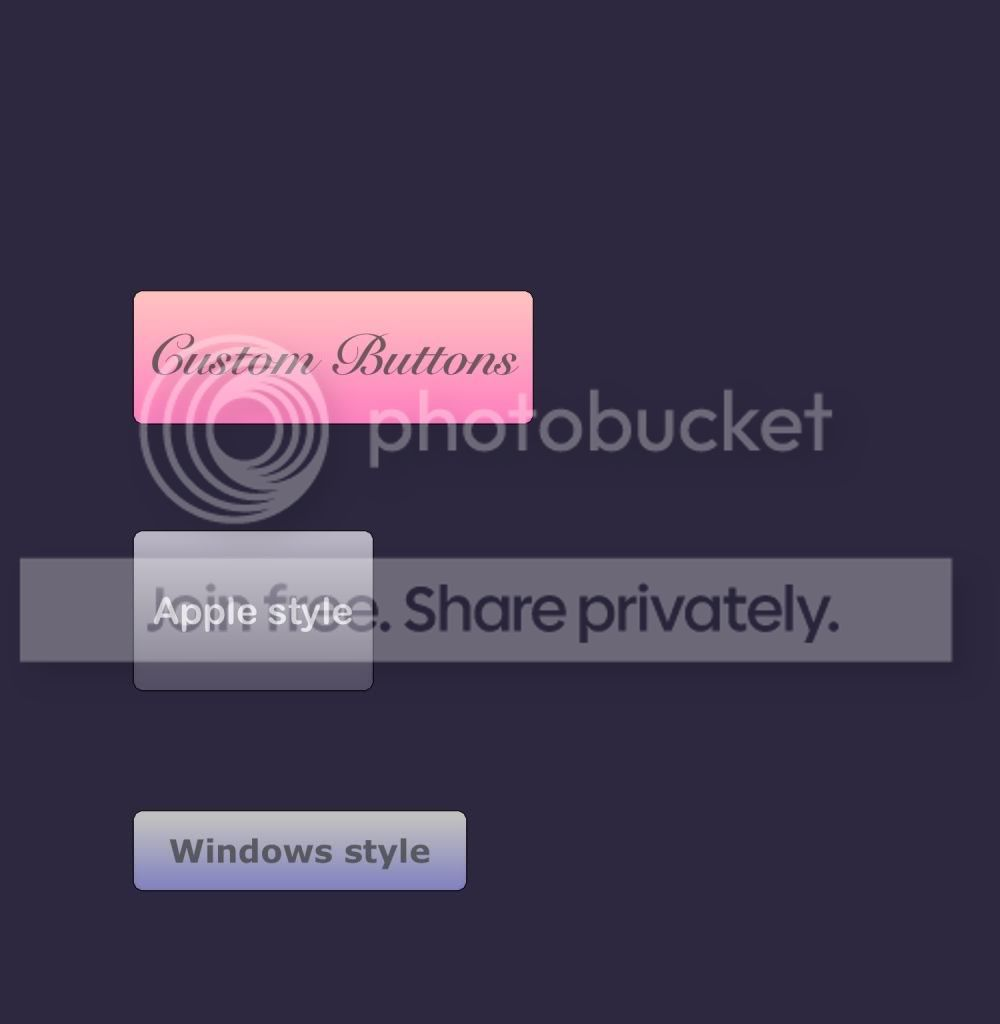#### Howdy, Stranger!

It looks like you're new here. If you want to get involved, click one of these buttons!

# Custom Button Control (beta)

edited May 2012 Posts: 179

I have been working to create a set of common controls for my codea projects, (i.e. buttons, toggle switches, status bars, etc.) and have a working button prototype. The event handler is started, but needs a little work to implement. It detects clicks, though, and gives visual feedback that it has been clicked (by way of changing colors).

It is made of a pretty simple mesh / gradient but I really like how it turned out. Hope someone finds it useful.``````button = class()
function button:init(text,location,width,height)
self.state = "normal"
self.text = text
self.textColor = color(255,255,255,192)
self.location = location
self.width = width
self.height = height
self.visible = true
self.fontSize = 28
self.font = "ArialRoundedMTBold"
self.color1 = color(255, 255, 255, 96)
self.color2 = color(128,128,128,32)
self.presscolor1 = color(192, 224, 224, 128)
self.presscolor2 = color(96, 192, 224, 128)
self.verts = self:createVerts(self.width, self.height)
self.myMesh = mesh()
self.myMesh.vertices = triangulate(self.verts)
self.vertColor = {}
self:recolor()
end
function button:setColors(c1,c2,p1,p2)
self.color1 = c1
self.color2 = c2
self.presscolor1 = p1
self.presscolor2 = p2
end
function button:textOptions(fn, sz, col)
self.font = fn
self.fontSize = sz
self.textColor = col
end
function button:draw()
if self.visible == true then
self:recolor()
pushMatrix()
translate(self.location.x,self.location.y)
self.myMesh:draw()
fill(self.textColor)
fontSize(self.fontSize)
font(self.font)
text(self.text, self.width/2,self.height/2)
self:drawLines(self.verts)
popMatrix()
end
end
function button:touched(touch)
if self.visible then
if touch.x >= self.location.x and touch.x <= self.location.x + self.width and touch.y >= self.location.y and touch.y <= self.location.y + self.height then
if touch.state == BEGAN then
self.state = "pressing"
elseif touch.state == ENDED then
if self.state == "pressing" then
self.state = "normal"
print "pressed."
end
end
else
self.state = "normal"
end
end
end
function button:createVerts(w,h)
local r
local v = {}
if w > 100 or h > 100 then
if w>=h then r = round(h/100) else r = round(w/100) end
else
r = 1
end
v = vec2(w,6*r)
v = vec2(w-r,4*r)
v = vec2(w-2*r,2*r)
v = vec2(w-4*r,r)
v = vec2(w-6*r,0)
v = vec2(6*r,0)
v = vec2(4*r,r)
v = vec2(2*r,2*r)
v = vec2(r,4*r)
v = vec2(0,6*r)
v = vec2(0,h-6*r)
v = vec2(r,h-4*r)
v = vec2(2*r,h-2*r)
v = vec2(4*r,h-r)
v = vec2(6*r,h)
v = vec2(w-6*r,h)
v = vec2(w-4*r,h-r)
v = vec2(w-2*r,h-2*r)
v = vec2(w-r,h-4*r)
v = vec2(w,h-6*r)
return v
end
function button:drawLines(v)
noSmooth()
strokeWidth(1)
stroke(0, 0, 0, 192)
for i=1, #v-1 do
line(v[i].x,v[i].y,v[i+1].x,v[i+1].y)
end
line(v[#v].x,v[#v].y,v.x,v.y)
end
function button:recolor()
local lt, dk
if self.state == "normal" then
lt = self.color1
dk = self.color2
else
lt = self.presscolor1
dk = self.presscolor2
end
for i=1,3 * #self.verts - 6 do
if self.myMesh.vertices[i].y > self.height/2 then
self.vertColor[i] = lt
else
self.vertColor[i] = dk
end
end
self.myMesh.colors = self.vertColor
end
function round(v)
return math.floor(v + 0.5)
end
``````

And the example program as pictured:

``````function setup()
b1 = button("Windows style", vec2(100, 100), 250, 60)
b1:setColors(color(192,192,192,255),color(128,128,192,255),
color(0,255,255,255),color(0,128,128,255))
b1:textOptions("Verdana-Bold", 24, color(64,64,64,192))
b2 = button("Apple style", vec2(100,250),180,120)
b3 = button("Custom Buttons", vec2(100, 450), 300, 100)
b3:setColors(color(255,192,192,255),color(255,128,192,255),
color(255, 92, 92, 255),color(255,0,0,255))
b3:textOptions("SnellRoundhand-Bold", 42, color(64,64,64,192))
end
function touched(touch)
b1:touched(touch)
b2:touched(touch)
b3:touched(touch)
end
function draw()
background(46, 39, 64, 255)
b1:draw();
b2:draw();
b3:draw();
end
``````
Tagged:

• Posts: 5,778

They look brilliant. Great use of mesh.

• Posts: 179

Thanks, @Simeon. I figure I will make a nice library of controls and then you will release Codea 1.8 or so with the full set of OS controls :-) Anyway, I appreciate the feedback. I'm not sure I have used any other programming tools with so much feedback and community interaction from the tool creators.

• Posts: 447

I'm def using this on my current project. Looks great!

• Posts: 447

quick bug fix: if w > 100 and h < 100, then r ends up being 0 in createVerts and that breaks triangulate. I'd fix with r = math.max(round(math.min(w,h)/100),1)

• Posts: 179

Thanks @ruilov. In the windows style example, the width is > 100 and height is < 100 and doesn't break there. I'm not exactly sure if thre is a bug or not, but your code is actually more concise anyway, so I will update. Thanks.

• edited May 2012 Posts: 447

@Vega, so this gave me the idea of coding a UI similar to the iOS settings screen. Here's the results. All the round buttons are using your code.

Code (edit: crappy, buggy, undocumented code)

https://github.com/ruilov/LIB-LUI

• Posts: 179

Nice, glad to see it in action. Btw, @ruilov, I have an idea for a game that will simulate hacking into secure computers using a terminal app, a port scanner, a password runner, etc. I am thinking That the windowing system you've been working on will be perfect for that job. Hope it is alright if I use it.

• Posts: 179

Whoops, that is actually @Deamos that has the windowing, sorry. But your controls you have there would be useful as well, if it is alright to use.

• Posts: 1,557

those are all very clean looking. I like!

• Posts: 447

@Vega of course!

• Posts: 122

@Vega, No problem with using the windowing system. Feel free to take it, change it, add to it, etc. Once I get my primary project done, I'm going to be iterating on the Windowing project to add some new stuff